﻿ 船厂钢板堆场混合存储分配及出入库调度研究
«上一篇文章快速检索 高级检索

 哈尔滨工程大学学报2017, Vol. 38Issue (11): 1786-1793  DOI: 10.11990/jheu.2016060590

### 引用本文HOU Jun, ZHANG Zhiying. Location allocation for inbound and outbound scheduling of mixed storage steel plate in shipyard[J]. Journal of Harbin Engineering University, 2017, 38(11): 1786-1793. DOI: 10.11990/jheu.201606059.### 文章历史

Location allocation for inbound and outbound scheduling of mixed storage steel plate in shipyard
HOU Jun, ZHANG ZhiyingSchool of Mechanical Engineering, Tongji University, Shanghai 200092, China
Abstract: To meet with the principle of managing shipyard that the steel plate in the stack yard is partly characterized by the block numbers and hybrid storage, this paper firstly verified that the assumption of mixed storage of block-oriented plates is feasible, and at the same time, optimized the shuffling costs followed by the outbound and inbound operations, and realized the purpose of reducing the costs including logistics and operation time of the processes, thereby improving the utilization of the stockyard. An integer programming model was formulated, and the approximately optimal solution was obtained according to the hierarchic genetic algorithm based on the simulated annealing probability accepted criterion (SA-HGA), programming the operation of pre-allocating for the two kinds of inbounding plates based on the current storage state, making sure that the plates inbounding is equally distributed, providing shuffling and out bounding plans according to the demand which costs less budget and time. At last, it's proved that the algorithm is of convergence and effectiveness by analyzing several cases, which are of different scales and make a contrast to the general genetic algorithm (GA). The results of the numerical experiments demonstrate the assumption and make an improvement to the operation plan of 4.9%~21.3% in cost and 7.6%~10.1% in operation time.
Key words: shipyard    stockyard    segment    steel plate operation scheduling    hierarchic genetic algorithm    simulated annealing

1 问题描述 1.1 问题背景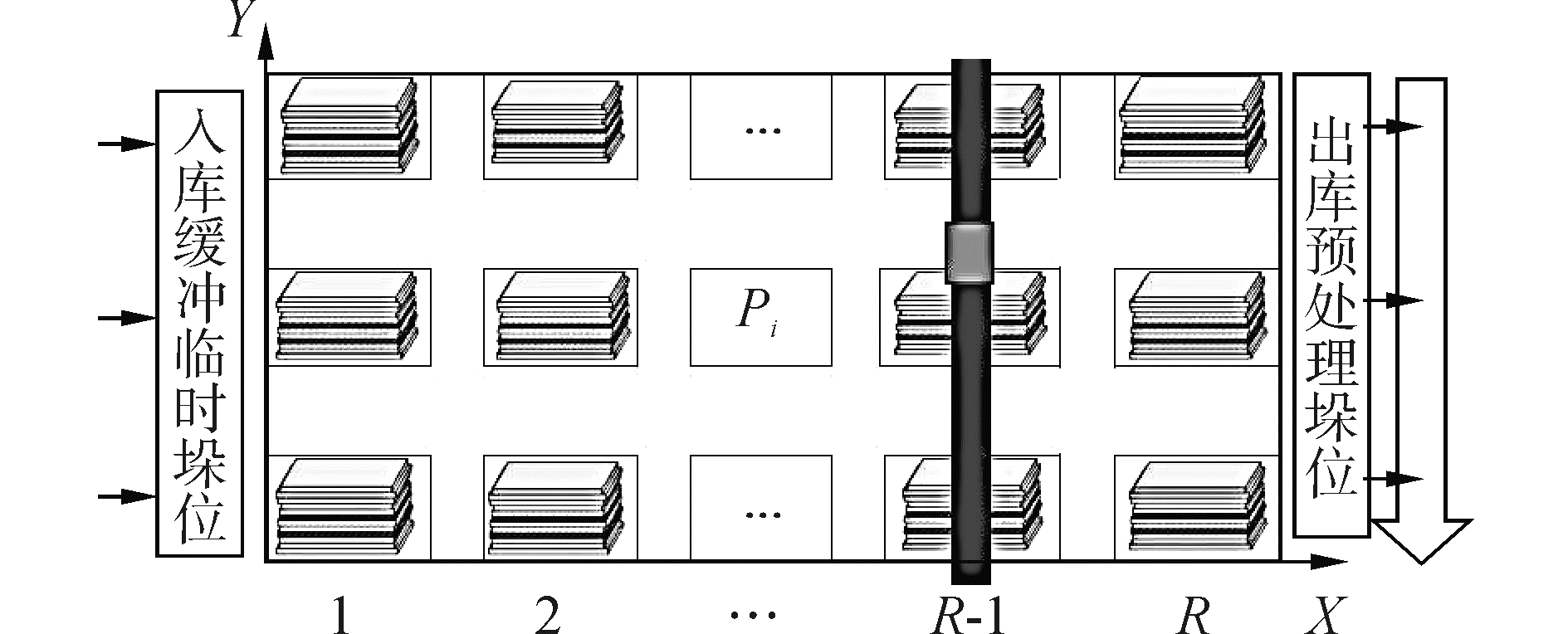图 1 堆场布局 Fig.1 Layout of stack yard

1) 主堆场垛位数量Y=RC，堆场以左上角为坐标原点，由一个桥吊承担出入库搬运作业；

2) 钢板从左端缓冲堆入库，进入堆场后由起始坐标搬运至预分配垛位；

3) 堆场每行(列)按照出库日期及尺寸划分，不考虑分段属性。从坐标原点开始，随着横(纵)坐标增大均匀分布；

4) 钢板可以在不同行(列)之间进行倒垛优先选择同列不同行，桥吊主体梁横向(或起重小车纵向)移动，且只考虑单张钢板搬运；

5) 假设堆场起始状态不为空，且以现有方式存储了一定量的钢板，并设垛位坐标Pi(Ci, Ri), (i=1, 2, …, Y)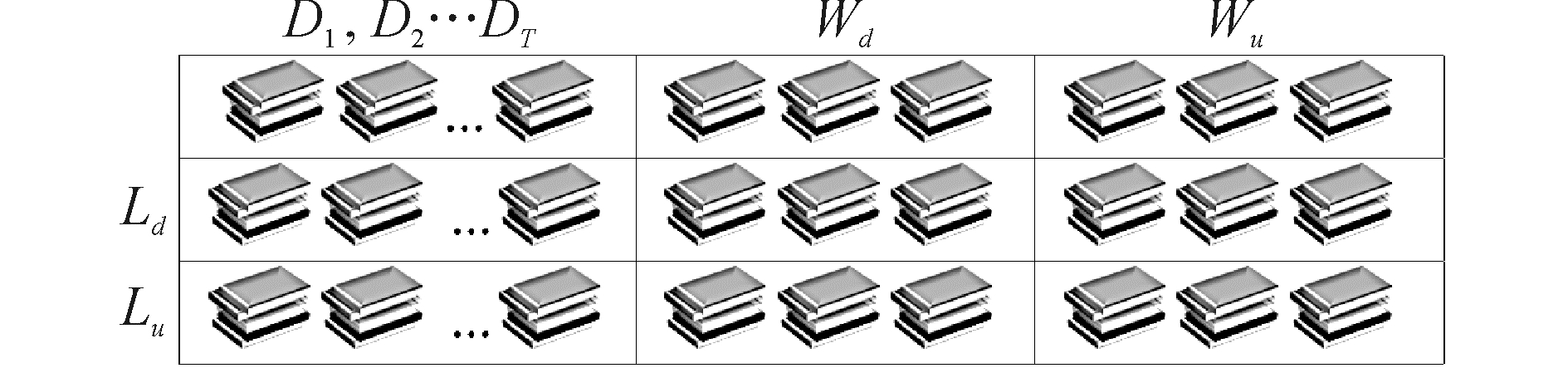图 2 堆场划分 Fig.2 Stack yard division

 ${f_i} = \iint\limits_{\left( {l,w} \right)d < \left( {l,w} \right)i \leqslant \left( {l,w} \right)u} {f{{\left( {l,w} \right)}_i},i \in \left[ {0,Y} \right]}$ (1)
 $\Delta f = \sqrt {\sum\limits_{i = 1}^Y {{{\left( {{f_i} - \mu } \right)}^2}} }$ (2)

1.2 出入库作业流程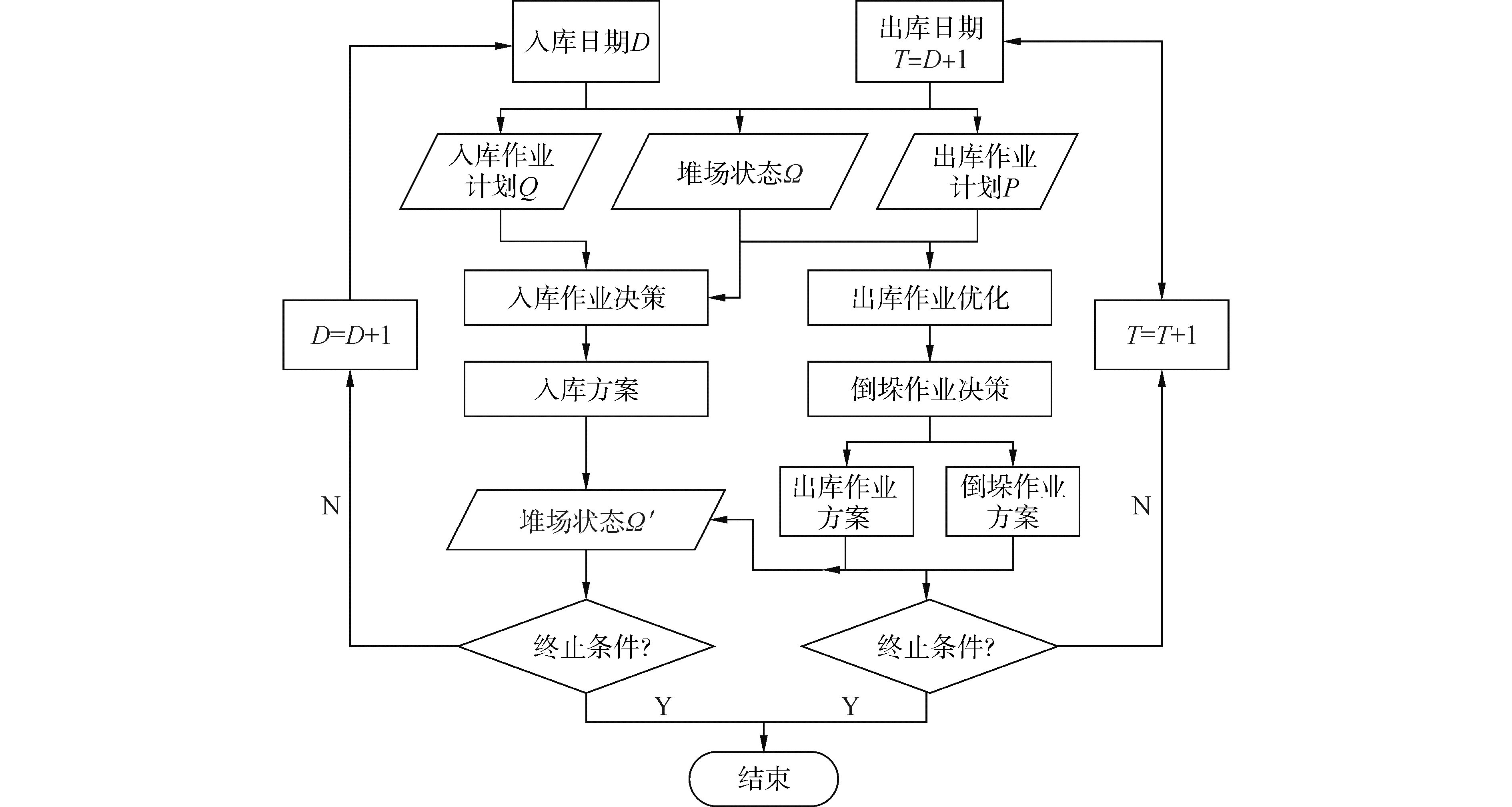图 3 钢板出入库流程 Fig.3 Logical flow of steel plate inbound and outbound

2 数学模型 2.1 堆垛及倒垛约束模型

 $\left| {{W_j} - {W_k}} \right| \le {W_m} + \left( {2 - {X_{ijr}} - {X_{ikr}} - 1} \right)Z$ (3)
 $\left| {{W_j} - {W_k}} \right| \le {W_m} + \left( {2 - {X_{ijr}} - {X_{ikr + 1}}} \right)Z$ (4)
 $\left| {{L_j} - {L_k}} \right| \le {L_m} + \left( {2 - {X_{ijr}} - {X_{ikr - 1}}} \right)Z$ (5)
 $\left| {{L_j} - {L_k}} \right| \le {L_m} + \left( {2 - {X_{ijr}} - {X_{ikr + 1}}} \right)Z$ (6)

 ${L_{\max t}} - {L_j} \le {\delta _{\max }} + \left( {1 - \sum\limits_{r - 1}^H {{X_{ijr}}} } \right)Z$ (7)
 $\begin{array}{*{20}{c}} {{L_j} - {L_{\min t}} \le {\delta _{\max }} + \left( {1 - \sum\limits_{r - 1}^H {{X_{ijr}}} } \right)Z}\\ {r = 1,2, \cdots ,{H_m}} \end{array}$ (8)

 $\begin{array}{*{20}{c}} {\left| {{{r'}_{ij}} - {r_{ij}}} \right| + \left| {{{c'}_{ij}} - {c_{ij}}} \right| \le 2,\left| {{{r'}_{ij}} - {r_{ij}}} \right| \le 2}\\ {\left| {{{c'}_{ij}} - {c_{ij}}} \right| \le 2} \end{array}$ (9)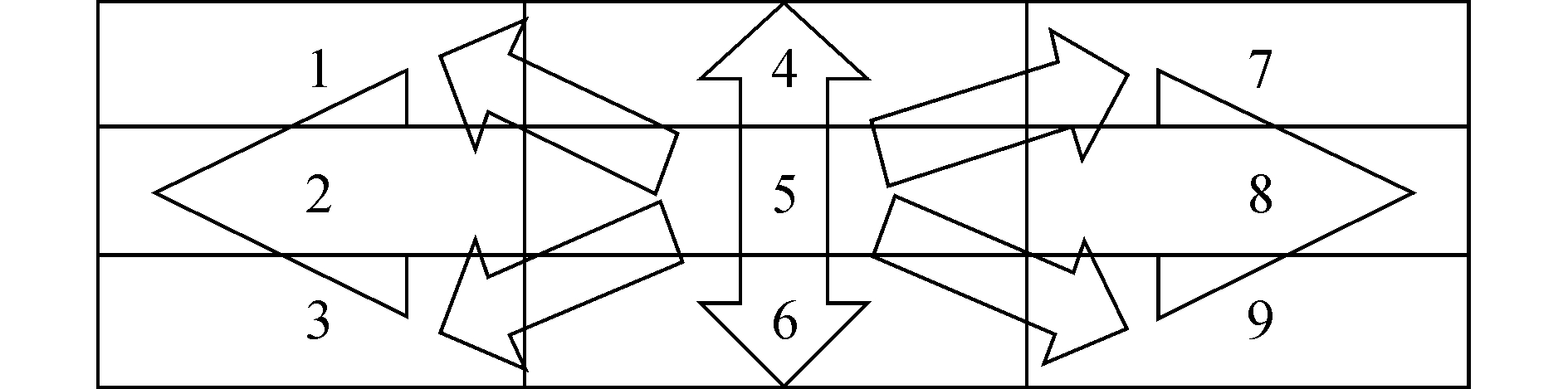图 4 相邻可倒垛位分布 Fig.4 Distribution of neighbored shuffling stack
2.2 目标函数及约束

 $M\;{\inf _1}\left( x \right) = \sum\limits_{i = 1}^M {\sum\limits_{j \in {S_i}} {{W_{ij}}{X_{ij}}} }$ (10)

 ${W_{ij}} = 2{t_c}\left( {{c_i} - {c_0}} \right) + {t_0} + \left( {{t_s} + {t_u}} \right){{D'}_{ij}}$ (11)

 $\begin{array}{*{20}{c}} {{D_{ij}} = \max \left\{ {{D_j} - \max \left\{ {{X_{ij}},{D_{j + 1}}\left| {I'} \right. = } \right.} \right.}\\ {\left. {\left. {1,2, \cdots ,\left( {i - 1} \right) \wedge {{P'}_{ij}} = {P_{ij}}} \right\},0} \right\}} \end{array}$ (12)

 $M\;{\inf _2}\left( {x,z} \right) = \sum\limits_{i = 1}^M {\sum\limits_{j \in Si} {{X_{ij}}\left\{ {{C_c}\left( {{c_{ij}} - {c_0}} \right) + {C_{{\rm{shuffle}}}}\left( {i,j} \right)} \right\}} }$ (13)

 $\begin{array}{*{20}{c}} {{C_{{\rm{shuffle}}}}\left( {i,j} \right) = \sum\limits_{k = {y_{ij}}}^{{K_m}} {\left\{ {\left( {{C_r}\left| {{{r'}_{ijk}} - {r_{ijk}}} \right| + } \right.} \right.} }\\ {\left. {\left. {2{C_c}\left| {{{c'}_{ijk}} - {c_{ijk}}} \right|} \right)} \right\}{Z_{ijk}}} \end{array}$ (14)

 $\sum\limits_{i = 1}^M {\sum\limits_{j \in Si} {\sum\limits_{k = {y_{ij}}}^{{k_m}} {{h_i}{X_{ij}}{Z_{ijk}}} } } \le H - h$ (15)

 $\sum\limits_{I = 1,j \in Si}^M {\sum\limits_{{A_j} = {y_i}} {{W_{i'j}}{X_{ij}}} } \le {T_i}$ (16)

 $\sum\limits_{j \in Si} {{X_{ij}} = {N_i},i = 1,2, \cdots ,M}$ (17)
 $\sum\limits_{i = 1}^M {{X_{ij}} \le 1} ,i = 1,2, \cdots ,M,j \in {U_i}$ (18)

3 模拟退火双层遗传算法 3.1 编码方式

1) 外层入库排序编码，设规格为ei的板入库NNi张，入库船板数共$NN=\sum\limits_{i=1}^{M}{N{{N}_{i}}}$。采用自然数编码方式，如图 5所示，按照编码对应自然数为入库集合包含的规格清单分配入库次序。图 5 入库编码 Fig.5 Inbound coding mode

2) 堆场状态Ω编码以及内层选择匹配组合出库编码，若堆场内钢板以数组堆栈存储，E[i][k](i∈[1, Y], k∈[1, Hm])。将符合规格ei的板设为i×10+1，十位以上为外层染色体出库次序编号。无板层设为0，有板层设为1，堆场可数字化为表 1所示。表 1 堆场垛位数字化编码 Tab.1 Digital en-coding mode for stack in stackyard

3) 设符合规格ei的钢板i对应的钢板集合Ui=(Pi1, Pi2, …Pij, …, Pini)，出库钢板候选板集合中元素个数$N=\sum\limits_{i=1}^{M}{{{n}_{i}}}$ni为钢板i可选集合Ui中元素个数，$U = \bigcup\limits_{i = 1}^M {{U_i}}$所有出库钢板的候选板集合；根据出库候选板集合Ui中元素个数ni初始化内层出库组合染色体，如图 6所示，采用0-1编码，1代表被选作对应出库板Pij，第i段为1的个数符合出库序列钢板Pi需求数量Ni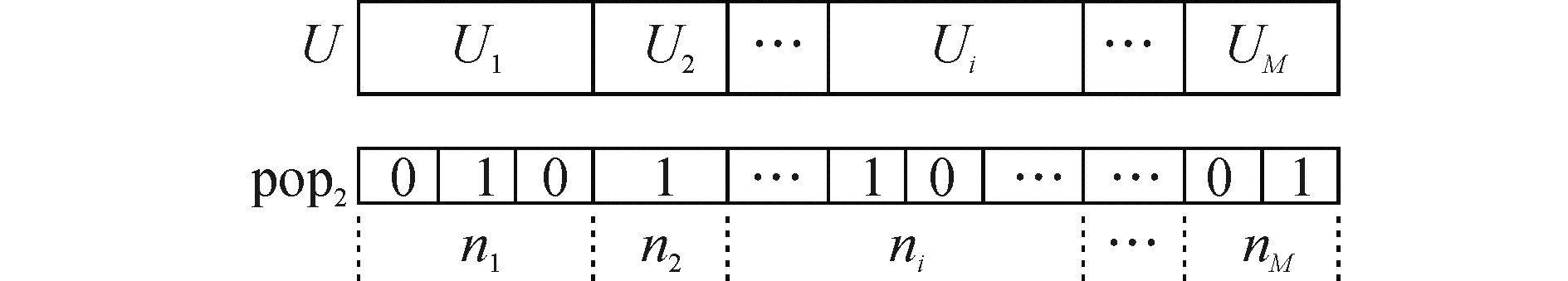图 6 出库组合编码 Fig.6 Outbound combining coding mode

4) 倒垛按步骤3染色体中为1的基因位确定目标板集合。倒垛集合也随之确定，为每块倒垛板建立可倒垛位集合。对于集合中每一个垛位，通过移动堆场状态编码中原位置和新位置的值反映钢板移动。每次移动令累积倒垛次数(shuffling number)SN=SN+1，最终在可倒垛集合中选择为完成该板出库作业预估倒垛量(SN值)最小的垛位组合。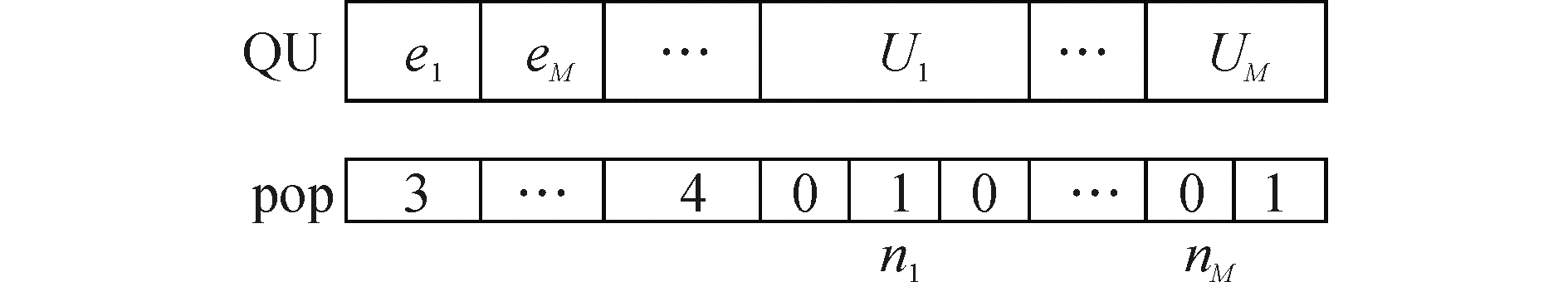图 7 GA染色体编码 Fig.7 GA chromosomecoding mode
3.2 算法流程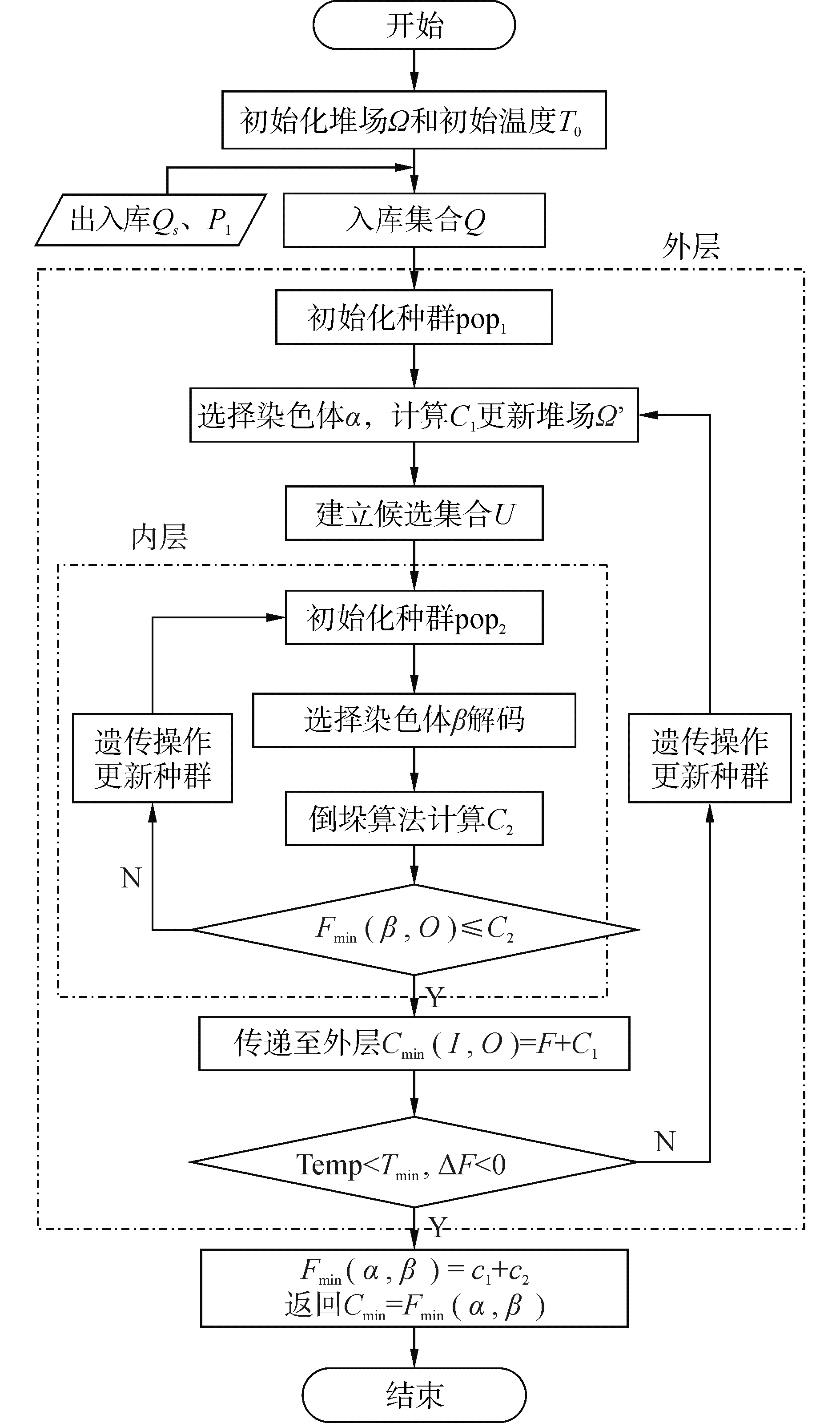图 8 模拟退火双层遗传算法流程 Fig.8 SA-HGA algorithm flow
4 实例验证与数值分析 4.1 参数设置表 2 初始参数设置 Tab.2 Original variables setting

4.2 实例验证与分析表 3 不指定分段与混合存储算例对比数据 Tab.3 Data comparison between mixed storage and non-segmented storage表 4 混合存储不同规模算例数据 Tab.4 Example in variable scales of mixed storage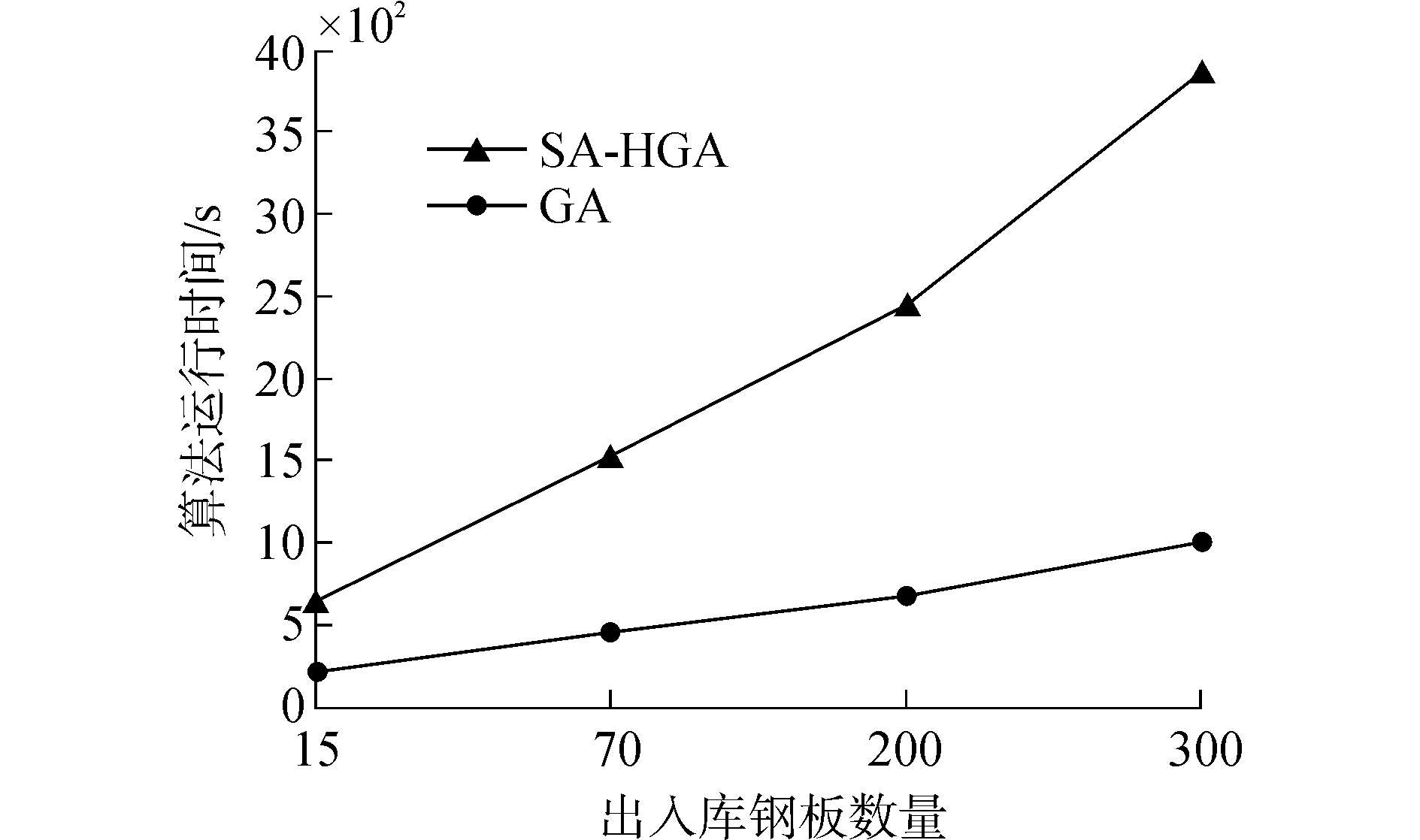图 9 算法运行时间随问题规模变化曲线图 Fig.9 Algorithm running time with variable problem scales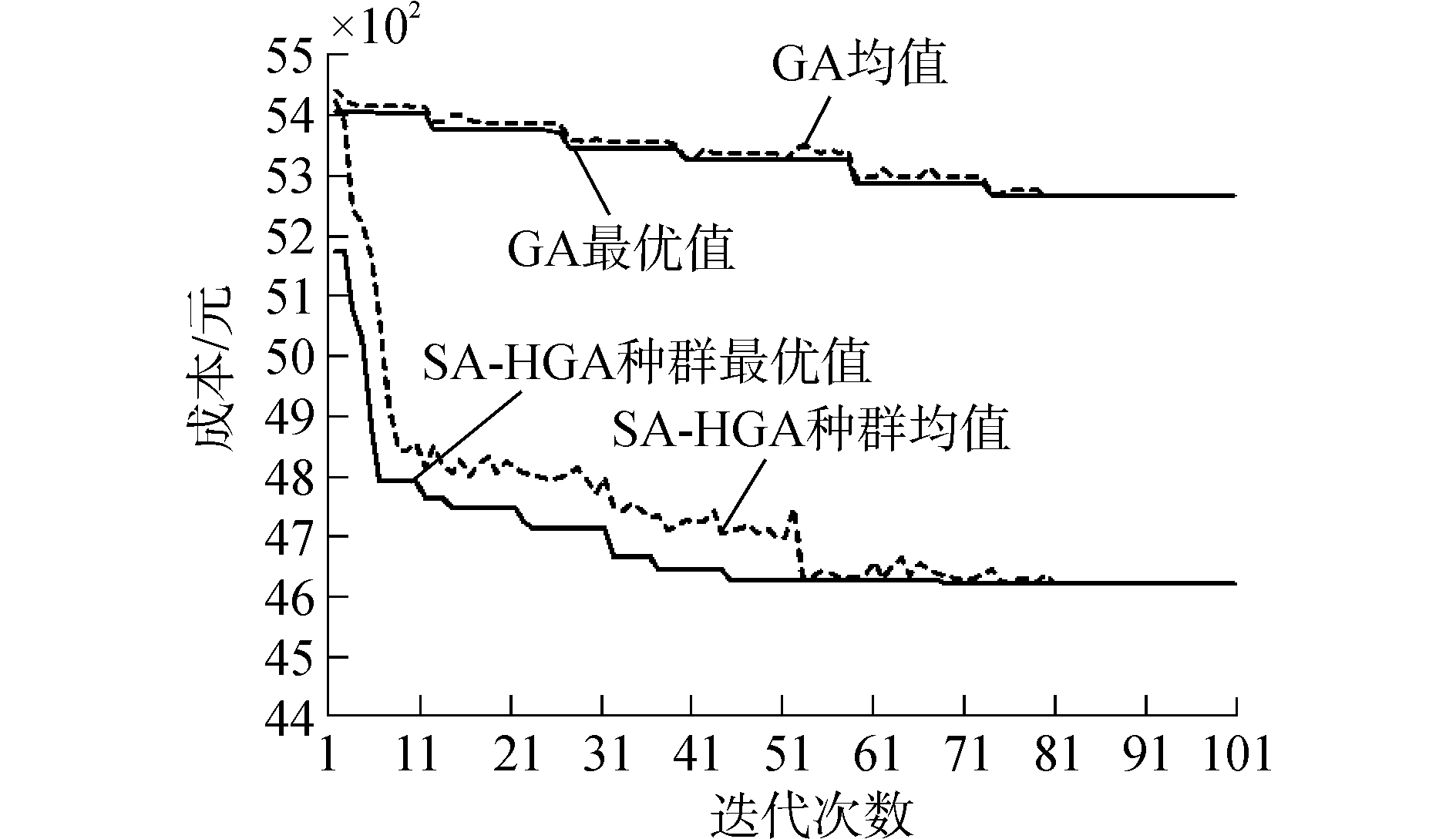图 10 SA-HGA和GA算法收敛性对比 Fig.10 Convergence performance of SA-HGA comparison to GA

5 结论

1) 提出了基于堆场当前状态，带分段属性和无分段属性两类钢板混合存储的方法，针对本文提出的多目标组合优化问题，建立了整数规划模型，进一步优化出入库作业成本和时间；

2)为适应于该多约束组合优化问题，提出运用模拟退火双层遗传算法优化作业计划，通过算法实例演算结果表明，算法能在一定的迭代次数内寻得较优解；提供堆场管理作业方案，大幅度降低堆场作业成本和时间、减少倒垛量并且具有较高的规模适应性。并与GA相比较，证明本文提出SA-HGA的可行性和有效性。

  施敏, 徐端, 刘宇晶. 垛位自动预约技术在宝钢船板配送中心库场管理系统中的应用[J]. 冶金自动化, 2011(6): 28-32. SHI Min, XU Duan, LIU Yujing. Applications of pile automatic appointment in BaosteelShip distribution center[J]. Metallurgical industry automation, 2011(6): 28-32. (0)  徐萍. 造船厂钢板入库作业优化及钢板管理信息系统开发[D]. 镇江: 江苏科技大学, 2011. XU Ping. Optimization on input operations and management information system development of a steel plate stacking yard in a shipyard[D]. Zhenjiang:Jiangsu University of Science and Technology, 2011. http://cdmd.cnki.com.cn/Article/CDMD-10289-1011289583.htm (0)  蒋如宏, 钟宏才, 谭家华. 船厂钢板堆场管理的数字化仿真[J]. 上海交通大学学报, 2003, 37(8): 1242-1245. JIANG Ruhong, ZHONG Hongcai, TAN Jiahua. Simulation of digitized plate stockyard management in shipyards[J]. Journal of Shanghai Jiao Tong University, 2003, 37(8): 1242-1245. (0)  刘建峰, 张小辉, 蒋志勇, 等. 基于遗传算法入库钢板分配策略研究[J]. 江苏科技大学学报:自然科学版, 2011(6): 524-529. LIU Jianfeng, ZHANG Xiaohui, JIANG Zhiyong, et al. Allocation strategy of steel plate in store house based on genetic algorithm[J]. Journal of Jiangsu University of Science and Technology:natural science edition, 2011(6): 524-529. (0)  唐立新, 杨自厚. 热轧实施计划中最优倒垛问题的整数规划模型及遗传算法[J]. 自动化学报, 2000, 26(4): 461-469. TANG Lixing, YANG Zihou. Integer programming model and modified genetic algorithm for optimalturned-out slab pile for hot rollinne schedule[J]. Acta automatic sinica, 2000, 26(4): 461-469. (0)  李耀华, 王伟, 胡国奋, 等. 热轧板坯库出库计划编制模型与算法研究[J]. 计算机集成制造系统, 2006, 12(3): 377-381. LI Yaohua, WANG Wei, HU Guofen, et al. Research on slab discharge planning model and algorithm for hot-rolling slab-yard[J]. Computer integrated manufacturing systems, 2006, 12(3): 377-381. (0)  ZHAO R, TANG L. Integer programming model and dynamic programming based heuristic algorithm for the heavy plate shuffling problem in the Iron and steel industry[C]//International Conference on Logistics Systems and Intelligent Management. 2010:1381-1385. http://ieeexplore.ieee.org/document/5461192/ (0)  ZHANG Z, WANG P, WANG W. Optimization and operation scheduling for a steel plate yard based on greedy algorithm[J]. Journal of networks, 2013, 8(7): 1654-1659. (0)  TANG L, REN H. Modelling and a segmented dynamic programming-based heuristic approach for the slab stack shuffling problem[J]. Computers & operations research, 2010, 37(2): 368-375. (0)  王广民. 造船厂钢板堆场出库作业计划建模及优化研究[D]. 大连: 大连理工大学, 2009. WANG Guangmin. Modeling and optimization on operation scheduling of a steel plate stacking yard in a shipyard[D]. Dalian:Dalian University of Technology, 2009. http://d.wanfangdata.com.cn/Thesis/Y1481136 (0)  张志英, 王维泽, 侯俊. 船厂钢板堆场多时段作业计划优化[J]. 哈尔滨工程大学学报, 2015(5): 638-643. ZHANG Zhiying, WANG Weize, HOU Jun. Optimization of multistage operation scheduling for a steel plate stockyard in shipbuilding[J]. Journal of Harbin Engineering University, 2015(5): 638-643. (0)  WANG G, JIN C, DENG X. Modeling and optimization on steel plate pick-up operation scheduling on stack yard of shipyard[C]//IEEE International Conference on Automation and Logistics, 2008:548-553. http://ieeexplore.ieee.org/document/4636211/ (0)